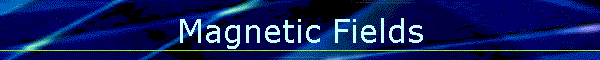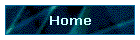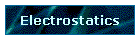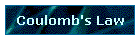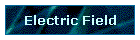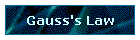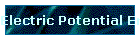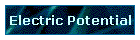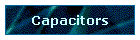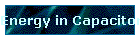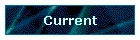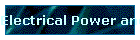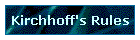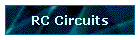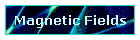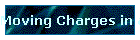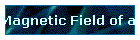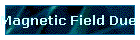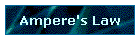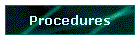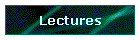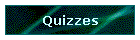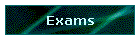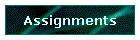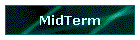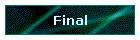Phys 4, Section 2                                                                 Feb 13, 2002   Magnetism (Permanent Magnets) Magnetism: first noticed as effect of iron ores by Greeks in Magnesia. Magnetic Poles: always N and S in a pair, never a separate monopole. Use of Magnet as compass Magnetic Field Magnetic field B is caused by moving charge (current); this field is experienced by other moving charges. F = qυ × B (Units of B are Tesla: 1 T = 1 N/A · m; also gauss: 1 G = 10-4 T) Lorentz Force: F = q(E + υ × B) Magnetic Field Lines and Magnetic Flux Field lines come out of N poles and go into S poles, except within the magnet itself where the lines go from S to N. Magnetic field lines have no origination or destination points, but always form closed loops. Magnetic flux is defined: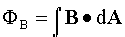Gauss' Law for Magnetism: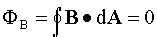Charged Particles in B Magnetic force F is at right angles to motion υ of charged particle q, so does no work, but can only change direction of motion, it acts as a centripetal force driving the particle in circular motion of radius R: F = qυB = mυ2/R but ω = υ/R so get  ω = qB/m (cyclotron frequency). Crossed B and E fields (balance of E-force and B-force): velocity selector: υ = E/B Thomson's e/m experiment: mv2/2 = eV → e/m = E2/2VB2 Magnetic Force on current-carrying conductor of length L:    F = IL × B       Last modified on February 27, 2002